# McDougal Littell Geometry Chapter 1: Basics of Geometry Chapter Exam

Exam Instructions:

Choose your answers to the questions and click 'Next' to see the next set of questions. You can skip questions if you would like and come back to them later with the yellow "Go To First Skipped Question" button. When you have completed the practice exam, a green submit button will appear. Click it to see your results. Good luck!

### Page 2

#### Question 7 7. What is shown here?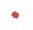#### Question 8 8. What is the relationship between Angle 4 and Angle 1?#### Question 9 9. Given the figure below, Angle 3 = 140y and Angle 5 = 80y + 70. Determine the value of y.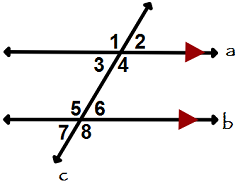#### Question 10 10. If angle 1 measures 70, what does angle 3 measure?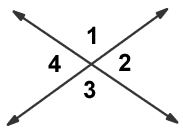### Page 3

#### Question 12 12. What is shown below?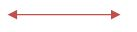#### Question 13 13. What is the relationship between Angle 1 and Angle 7?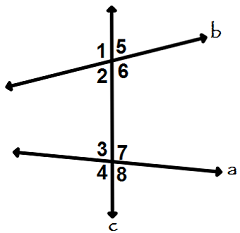### Page 4

#### Question 19 19. What is shown below?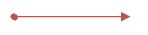### Page 5

#### Question 24 24. In the sequence 9, 14, 19, 24, 29,.... Say we're using a to describe the terms. What is the value of the term below?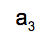#### Question 25 25. What is the midpoint of this line segment?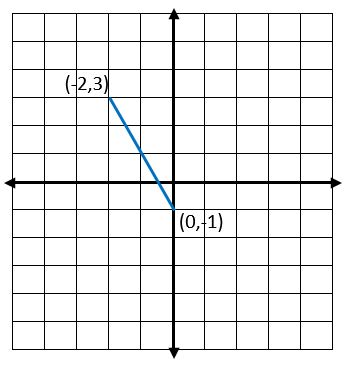### Page 6

#### Question 27 27. Consider the figure below, where Angle 4 = 12m - 4 Angle 5 = 10m + 20 Calculate the measure of Angle 5.#### McDougal Littell Geometry Chapter 1: Basics of Geometry Chapter Exam Instructions

Choose your answers to the questions and click 'Next' to see the next set of questions. You can skip questions if you would like and come back to them later with the yellow "Go To First Skipped Question" button. When you have completed the practice exam, a green submit button will appear. Click it to see your results. Good luck!

Support